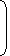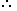# Tips and Tricks To Solve Problems on Races and Games1. Races: A contest of speed in running, riding, driving, sailing or rowing is called a race.
2. Race Course: The ground or path on which contests are made is called a race course.
3. Starting Point: The point from which a race begins is known as a starting point.
4. Winning Point or Goal: The point set to bound a race is called a winning point or a goal.
5. Winner: The person who first reaches the winning point is called a winner.
6. Dead Heat Race: If all the persons contesting a race reach the goal exactly at the same time, the race is said to be dead heat race.
7. Start: Suppose A and B are two contestants in a race. If before the start of the race, A is at the starting point and B is ahead of A by 12 metres, then we say that ‘A gives B, a start of 12 metres’.To cover a race of 100 metres in this case, A will have to cover 100 metres while B will have to cover only (100 – 12) = 88 metres.In a 100 race, ‘A can give B 12 m’ or ‘A can give B a start of 12 m’ or ‘A beats B by 12 m’ means that while A runs 100 m, B runs (100 – 12) = 88 m.
8. Games: ‘A game of 100, means that the person among the contestants who scores 100 points first is the winner’.If A scores 100 points while B scores only 80 points, then we say that ‘A can give B 20 points’.
1. In a 100 m race, A can give B 10 m and C 28 m. In the same race B can give C:
 A. 18 m B. 20 m C. 27 m D. 9 m

Answer: Option B

Explanation:

A : B = 100 : 90.

A : C = 100 : 72.

 B : C = B x A = 90 x 100 = 90 . A C 100 72 72

When B runs 90 m, C runs 72 m.

 When B runs 100 m, C runs72 x 100m = 80 m. 90B can give C 20 m.

2. A and B take part in 100 m race. A runs at 5 kmph. A gives B a start of 8 m and still beats him by 8 seconds. The speed of B is:
 A. 5.15 kmph B. 4.14 kmph C. 4.25 kmph D. 4.4 kmph

Answer: Option B

Explanation:

 A’s speed =5 x 5m/sec = 25 m/sec. 18 18
 Time taken by A to cover 100 m =100 x 18sec = 72 sec. 25Time taken by B to cover 92 m = (72 + 8) = 80 sec.B’s speed =92 x 18kmph = 4.14 kmph. 80 5

#### In a 500 m race, the ratio of the speeds of two contestants A and B is 3 : 4. A has a start of 140 m. Then, A wins by:

Correct! Wrong!

#### In a 100 m race, A beats B by 10 m and C by 13 m. In a race of 180 m, B will beat C by:

Correct! Wrong!

#### At a game of billiards, A can give B 15 points in 60 and A can give C to 20 points in 60. How many points can B give C in a game of 90?

Correct! Wrong!

#### In a race of 200 m, A can beat B by 31 m and C by 18 m. In a race of 350 m, C will beat B by:

Correct! Wrong!

#### In 100 m race, A covers the distance in 36 seconds and B in 45 seconds. In this race A beats B by:

Correct! Wrong!

QUIZ ON RACES & GAMES
GREAT JOB!!!!

Share your Results:

Don't miss out!
Subscribe To Our Newsletter

Learn new things. Get an article everyday.

Invalid email address
Give it a try. You can unsubscribe at any time.